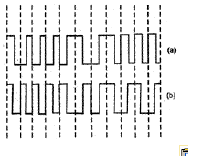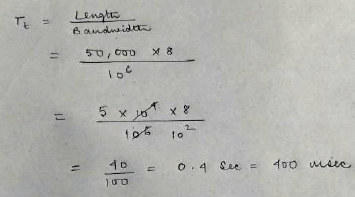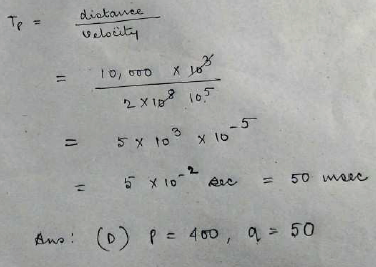Courses

# Network & Its Security

## 10 Questions MCQ Test GATE Computer Science Engineering(CSE) 2022 Mock Test Series | Network & Its Security

Description
This mock test of Network & Its Security for Computer Science Engineering (CSE) helps you for every Computer Science Engineering (CSE) entrance exam. This contains 10 Multiple Choice Questions for Computer Science Engineering (CSE) Network & Its Security (mcq) to study with solutions a complete question bank. The solved questions answers in this Network & Its Security quiz give you a good mix of easy questions and tough questions. Computer Science Engineering (CSE) students definitely take this Network & Its Security exercise for a better result in the exam. You can find other Network & Its Security extra questions, long questions & short questions for Computer Science Engineering (CSE) on EduRev as well by searching above.
QUESTION: 1

### In Ethernet when Manchester encoding is used, the bit rate is:

Solution:

Bit rate is half the baud rate in Manchester encoding as bits are transferred only during a positive transition of the clock.

QUESTION: 2

### In the waveform (a) given below, a bit stream is encoded by Manchester encoding scheme. The same bit stream is encoded in a different coding scheme in wave form (b). The bit stream and the coding scheme areSolution:

THE AMBIGUOUS QUESTION WITHOUT ANY STANDARD MENTION !!
BOTH A and B is correct , it is just the convention which determine the correct answer.

as per IEEE B is correct
as per G E Thomas A is correct.
as we ususally follow IEEE thus B is correct

QUESTION: 3

### The address resolution protocol (ARP) is used for:

Solution:
QUESTION: 4

Consider two hosts X and y, connected by a single direct link of rate 106 bits/sec. The distance between the two hosts is 10,000 km and the propagation speed along the link is 2x108 m/sec. Host X sends a file of 50,000  bytes as one largemessage to host Y continuously. Let the transmission and propagation delays be  p milliseconds and q millisecondsrespectively. Then the value of p and q are

Solution:Hence answer d

QUESTION: 5

(v) A simple and reliable data transfer can be accomplished by using the 'handshake protocol'. It accomplishes reliable data transfer because for every data item sent by the transmitter _____.

Solution:
QUESTION: 6

02. Choose the correct alternatives (more than one may be correct) and write the corresponding letters only: (v). Start and stop bis don not contain an 'information' but are used in serial communication

Solution:

Answer: C
The start and stop bits are used to synchronize the serial receivers.

QUESTION: 7

In a data link protocol, the frame delimiter flag is given by 0111. Assuming that bit stuffing is employed, the transmitter sends the data sequence 01110110 as

Solution:

Answer will be option D.)
The bit stuffing is done after every two '11' (as flag is 0111) to differentiate the data part from the flag- there must not be
"111" in the data so after every 11 a '0' is added. The receiver also knows this and so it decodes every "110" as "11".
Therefore option D. is the answer.

QUESTION: 8

A TCP message consisting of 2100 bytes is passed to IP for delivery across two networks. The first network can carry amaximum payload of 1200 bytes per frame and the second network can carry a maximum payload of 400 bytes per frame,excluding network overhead. Assume that IP overhead per packet is 20 bytes. What is the total IP overhead in the secondnetwork for this transmission?

Solution:

Answer is C).
Question says TCP message is of size = 2100 B, hence header is encapsulated with the frame and send to IP layer, and IP
layer when receives this message thinks it as complete data to be send .
To be send across the first network, It is fragmented into 2 payloads of sizes 1200 and 900 .
No need to add IP Header size with 2100B, as fragmentation acts on data/Payload and not the packet.
Now, since, IP thinks 2100B as the data so, it will directly fragment it and hence, no need to add any header.
Payload / Fragment 1: 1200 B {1200 / 8 = 150, Except last fragment all the fragments should be divisible by 8}
Payload 2: 900 B
At the second network, 1200 B is fragmented into 400 B,400 B and 400 B .
Similarly, 900 B is fragmented into 400, 400 and 100 B.
Since, 2100 B is fragmented into 6 data payloads and Header is attached to all the payloads when they will be forwared to
DLL layer. So, Total Overhead = 6 * 20 = 120 B
Hence, DLL layer will receive total Data = 2100 + 120 = 2220 B and DLL will treat it as a complete data to be sent and
DLL header will be encapsulated in DLL frame.

QUESTION: 9

A channel has a bit rate of 4 kbps and one-way propagation delay of 20 ms. The channel uses stop and wait protocol. Thetransmission time of the acknowledgement frame is negligible. To get a channel efficiency of at least 50%, the minimumframe size should be

Solution:

for 50% utilization
tt/(tt+2tp)>=1/2
2tt>=tt+2tp

tt>=2tp
L/B>=2*tp
L>=2*tp*B
so here L=2*20*10^-3*4*10^3
=160 bits
so ans is d

QUESTION: 10

A link of capacity 100 Mbps is carrying traffic from a number of sources. Each source generates an on-off traffic stream;
when the source is on, the rate of traffic is 10 Mbps, and when the source is off, the rate of traffic is zero. The duty cycle,
which is the ratio of on-time to off-time, is 1 : 2. When there is no buffer at the link, the minimum number of sources that can be multiplexed on the link so that link capacity is not wasted and no data loss occurs is S1. Assuming that all sources are synchronized and that the link is provided with a large buffer, the maximum number of sources that can be multiplexed so that no data loss occurs is S2. The values of S1 and S2 are, respectively,

Solution:

Since there is no buffer.. and constraint given is there should not be any data lost, and no wastage of capacity as well..
Since data should not be lost, we calculate for the extreme case when all sources are on-time (that is transmitting)..
10 Mbps * n-station 100 Mbps
n-station = 10..
In the next part of the question it is given that the link is provided with large buffer and we are asked to find out large no. of stations..
for that we'll calculate expected value of bandwidth usage (if more data comes we store in buffer and due to expectation,
the buffer will be emptied soon):
E = 1/3 * 10 + 1/3 * 10 +...n-station times 100 Mbps [ total time is (1+2) = 3 then on time is 1 so 1/3 of BW]
=> 1/3 * 10 * n-station 100 Mbps
=> n-station = 30
so, option (A)